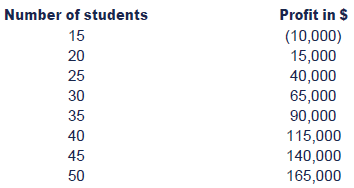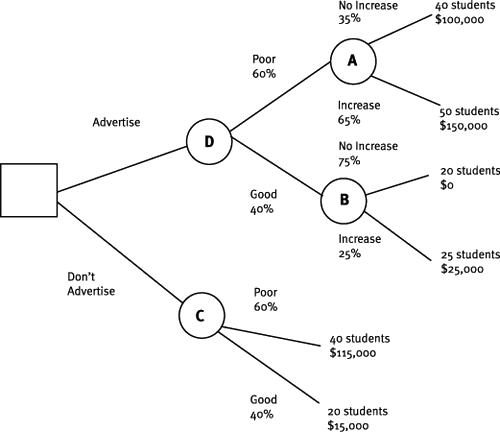ACCAPEDIA

Kaplan Wiki > ACCAPEDIA > Wiki Pages > Decision Trees

Decision TreesFavorite|Discussions
| |History
|Quick Launch View All Site ContentDecision Trees

Back up a level
 Contents [Hide]

Decision Trees

Decision trees are used in the context of decision making under uncertainty and are a useful tool for seeing the various possible outcomes of different decisions

Decision trees

A decision tree is a diagrammatic representation of a multi-decision problem, where all possible courses of action are represented, and every possible outcome of each course of action is shown.

Decision trees should be used where a problem involves a series of decisions being made and several outcomes arise during the decision-making process. Decision trees force the decision maker to consider the logical sequence of events. A complex problem is broken down into smaller, easier to handle sections.

The financial outcomes and probabilities are shown separately, and the decision tree is 'rolled back' by calculating expected values and making decisions.

Method

Step 1: Draw the tree from left to right, showing appropriate decisions and events / outcomes.

Some common symbols can be used:

• a square is used to represent a decision point (i.e. where a choice between different courses of action must be taken.
• A circle is used to represent a chance point.
• The branches coming away from a circle with have probabilities attached to them. All probabilities should add up to '1'.

Label the tree and relevant cash inflows/outflows and probabilities associated with outcomes.

Step 2: Evaluate the tree from right to left carrying out these two actions:

(a) Calculate an expected value (EV) at each outcome point.

(b) Choose the best option at each decision point.

Step 3: Recommend a course of action to management.

Illustration - Decision trees

A university is trying to decide whether or not to advertise a new post-graduate degree programme.

The number of students starting the programme is dependent on economic conditions:

• If conditions are poor it is expected that the programme will attract 40 students without advertising. There is a 60% chance that economic conditions will be poor.
• If economic conditions are good it is expected that the programme will attract only 20 students without advertising. There is a 40% chance that economic conditions will be good.

If the programme is advertised and economic conditions are poor, there is a 65% chance that the advertising will stimulate further demand and student numbers will increase to 50. If economic conditions are good there is a 25% chance the advertising will stimulate further demand and numbers will increase to 25 students.

The profit expected, before deducting the cost of advertising, at different levels of student numbers are as follows:Required:

Demonstrate, using a decision tree, whether the programme should be advertised.

Step 1: Draw the tree from left to right. A square is used to represent a decision point (i.e. whether to advertise the programme, or not advertise.).

For both options, a circle is used to represent a chance point - a poor economic environment, or a good economic environment.

Label the tree and relevant cash inflows/outflows and probabilities associated with outcomes :Step 2: Evaluate the tree from right to left carrying out these two actions:

(a)   Calculate an Expected Value at each outcome point. Working from top to bottom, we can calculate the EVs as follows:

• EV (Outcome Point A) = (35% x \$100,000) + (65% x \$150,000) = \$132,500
• EV (Outcome Point B) = (0% x \$0) + (25% x \$25,000) = \$6,250
• EV (Outcome Point C) = (60% x \$115,000) + (40% x \$15,000) = \$75,000
• EV (Outcome Point D) = (60% x \$132,500) + (40% x \$6,250) = \$82,000

(b)   Choose the best option at each decision point and recommend a course of action to management.

At the first (and only) decision point in our tree, we should choose the option to advertise as EV ('D') is \$82,000 and EV ('C) is \$75,000.

Further complications

A further complication that arises with decision trees is that they allow you to calculate the value of having further information, say about market conditions, which in turn allows you to decide whether or not it is worth paying for market research. In exam questions this is often split between perfect and imperfect information.Created at 7/10/2012 11:26 AM  by System Account  (GMT) Greenwich Mean Time : Dublin, Edinburgh, Lisbon, London Last modified at 9/26/2013 2:38 PM  by System Account  (GMT) Greenwich Mean Time : Dublin, Edinburgh, Lisbon, LondonRating :Ratings & Comments  (Click the stars to rate the page)Tags:Recent Discussions

 There are no items to show in this view.ACCAPEDIA - Decision Trees